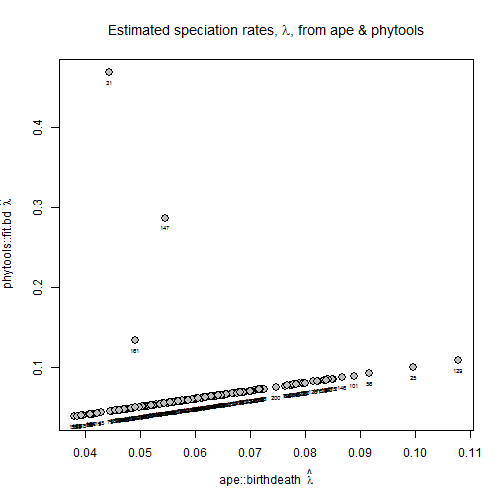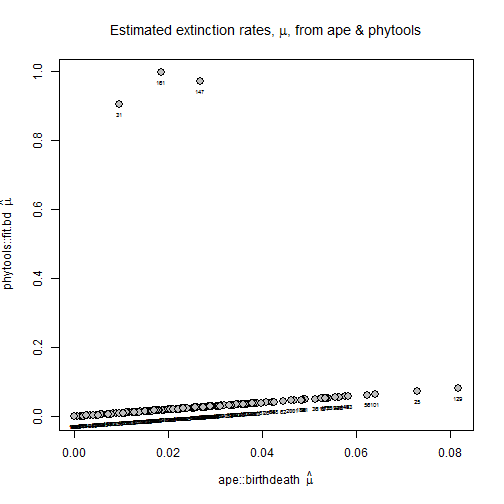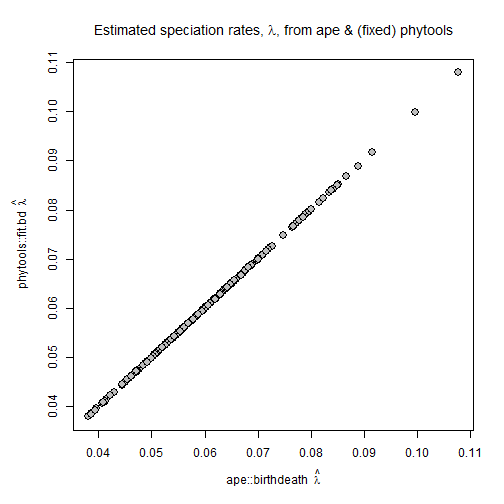## Sunday, October 29, 2017

### Fix to optimization routine for non-finite log-likelihoods in `fit.bd`

I recently added the function `fit.bd` to theh phytools package. This function fits a birth-death model & essentially duplicates existing function in ape and diversitree.

I discovered, however, that sometimes optimization fails even for this simple model, and it seems to be usually due to numerical issues which causes the log-likelihood to evaluate to a non-finite value during optimization. This can result in some weird discrepancies between optimized birth (λ) and death (μ) rates between phytools and ape.

This is what I mean:

``````library(phytools)
library(TreeSim)
set.seed(1)
trees<-sim.bd.taxa.age(n=100,numbsim=200,lambda=0.059,
mu=0.020,frac=1,age=100,mrca=TRUE)
fits<-lapply(trees,fit.bd)
``````
``````## There were 9 warnings (use warnings() to see them)
``````
``````## birth & death rates from ape
BD.ape<-t(sapply(trees,function(x) bd(birthdeath(x))))
## birth & death rates from phytools
BD.phytools<-t(sapply(fits,function(x)
setNames(c(x\$b,x\$d),c("b","d"))))
plot(BD.ape[,"b"],BD.phytools[,"b"],cex=1.5,pch=21,bg="grey",
xlab=expression(paste("ape::birthdeath  ",hat(lambda))),
ylab=expression(paste("phytools::fit.bd  ",hat(lambda))))
text(BD.ape[,"b"],BD.phytools[,"b"],1:length(fits),cex=0.5,pos=1)
title(main=expression(paste("Estimated speciation rates, ",lambda,
", from ape & phytools")))
````````````plot(BD.ape[,"d"],BD.phytools[,"d"],cex=1.5,pch=21,bg="grey",
xlab=expression(paste("ape::birthdeath  ",hat(mu))),
ylab=expression(paste("phytools::fit.bd  ",hat(mu))))
text(BD.ape[,"d"],BD.phytools[,"d"],1:length(fits),cex=0.5,pos=1)
title(main=expression(paste("Estimated extinction rates, ",mu,
", from ape & phytools")))
``````We can immediately see that three trees, #31, #147,& #161, have estimated speciation & extinction rates that are way off!

If we look at their log-likelihoods it turns out they are all non-finite:

``````sapply(fits[c(31,147,161)],logLik)
``````
``````##  Inf Inf Inf
``````

The simple fix that I have pushed so far is to simply check if the optimized log-likelihood is finite, and then, if not, to re-run the optimization routine with different starting values (or until a certain number of iterations is reached).

Let's load it & try it. (Note that the user should just update phytools from GitHub).

``````source("https://raw.githubusercontent.com/liamrevell/phytools/master/R/bd.R")
qb<-phytools:::qb ## make internal function visible
fits<-lapply(trees,fit.bd)
``````
``````## There were 32 warnings (use warnings() to see them)
``````
``````b<-sapply(fits,function(x) x\$b)
par(mar=c(5.1,5.1,4.1,2.1))
plot(BD.ape[,"b"],b,cex=1.5,pch=21,bg="grey",
xlab=expression(paste("ape::birthdeath  ",hat(lambda))),
ylab=expression(paste("phytools::fit.bd  ",hat(lambda))))
title(main=expression(paste("Estimated speciation rates, ",lambda,
", from ape & (fixed) phytools")))
``````Good!